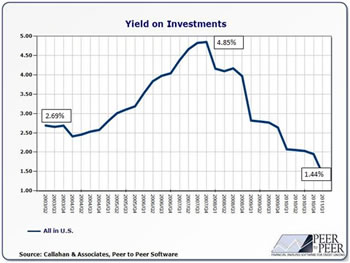# Difference between Yield and Interest Rate

##### Key difference: A striking difference between a yield and an interest rate is that yield is the profit made on an investment, and an interest rate is the reason behind such a profit.Interest rate and yield are two terms commonly used by banks, financial firms, brokers, investment funds, etc., for luring investors into their manifold schemes. While investing in a commodity, it is imperative that an investor brushes up his knowledge about what is actually meant by these financial jargons, which are rapidly thrown up at dime a dozen.

Yield can be understood as the collective profit earned by investing in financial commodities like shares, debentures, bonds, etc. Yield is more accurate and gives a precise understanding of the total earning made from an investment. The reason behind this is that yield also takes into consideration factors such as tax benefits. To understand how yield works, one has to first delve into the concept of an interest rate.

An interest rate is nothing but the percentage of amount gained or paid, on a principal amount. In case of income investments such as fixed deposits, recurring deposits, etc., the rate of interest signifies the percentage of amount to be received, over the initial investment made. While in terms of borrowing or lending loans, an interest rate means the percentage of amount to be paid by the borrower to the lender, over the total amount borrowed.

Yield can be calculated only after taking the interest rate into consideration. For instance, if an investor has invested \$1000 in a dividend at an interest rate of 5% compounded quarterly, the yield of this investment can only be arrived at after calculating the interest earned. The interest on this investment would be \$50 for the first quarter, but second quarter onwards, interest would be gained on a collective amount obtained by adding the previous interest to the principal amount, i.e. \$1050 and so on.As a year has four quarters, the interest would be calculated four times, and the total amount gained over the year adding all the other monetary benefits such as tax, will be the total yield on this investment. A notable feature of yield is that it is always calculated annually, i.e., on a per annum basis, and often expressed in terms of percentage. Interest can be calculated yearly, half yearly, quarterly, monthly, etc.

Therefore, it abides on an investor to draw a line of understanding between a yield and an interest rate, and then proceed towards making an investment.

Comparison between Yield and Interest rate:

 Yield Interest rate Meaning Yield is the total earning made on an investment, including the interest. Interest rate is the percentage of amount to be gained or paid, over a principal amount. Period of calculation Yield is always annually calculated. Interest can be calculated annually, monthly, quarterly, half-yearly, etc. Vis-à-vis Yield is always higher than an interest. Interest is always lower than yield. Expression Yield can be expressed as percentage and as amounts of currency as well. Interest rates are largely expressed in terms of percentage. Overlapping Yield always includes the amount of interest gained. Interest is calculated independent of yield.

Image Courtesy: snbchf.com, thingsboganslike.com